# Silicon tetrachloride (SiCl4) lewis dot structure, molecular geometry, polar or non-polar, hybridization

Home  > Chemistry Article > SiCl4 lewis structure and its molecular geometry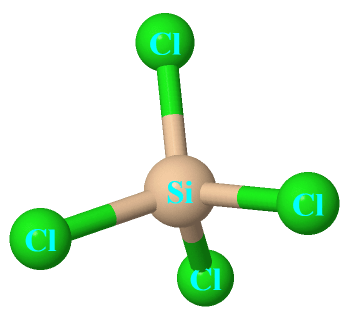Silicon tetrachloride is an inorganic compound that appears as a colorless liquid with a pungent odor having the chemical formula SiCl4. It can react with water violently and forms white solid silicon dioxide and HCl gas. It is soluble in water.

In this article, we will discuss Silicon tetrachloride(SiCl4) lewis structure, molecular geometry, hybridization, polar or nonpolar, etc.

Silicon tetrachloride is corrosive to tissue and metal. It is used to produce high-quality silica for commercial purposes. High purity of silicon tetrachloride used in the manufacture of optical fibers.

Properties of Silicon tetrachloride

• It is soluble in benzene, toluene, and water.
• It appears as a colorless fuming liquid.
• It has a boiling point of 57.65 °C and a melting point of −68.74 °C.
• It has a tetrahedral crystal structure.
• The coordination geometry number of SiCl4 is 4.
 Name of Molecule Silicon tetrachloride Chemical formula SiCl4 Molecular geometry of SiCl4 Tetrahedral Electron geometry of SiCl4 Tetrahedral Hybridization Sp³ Bond angle 109.5º Total Valence electron for SiCl4 32
Page Contents

## How to draw lewis structure for SiCl4?

SiCl4 lewis’s structure is straightforward and very easy to draw. “Lewis diagram describes the chemical bonding of atoms within a molecule”. Lewis structure of SiCl4 contains 12 lone pairs on surrounding atoms and zero on the central atom.

There are 4 bonding pairs present in the lewis structure of Silicon tetrachloride. Let’s see how to draw this step by step-

## Follow some steps for drawing the lewis dot structure of SiCl4

1. Count total valence electron in SiCl4

First of all, find the availability of valence electrons for SiCl4, for this, just find the valence electron of silicon and chlorine atoms through the periodic group number.

As silicon belongs to the 14th periodic group and chlorine to the 17th group. Therefore, the valence electron for silicon is 4 and for chlorine, it is 7.

⇒ Total number of the valence electrons in silicon = 4

⇒ Total number of the valence electrons in chlorine = 7

∴ Total number of valence electron available for the lewis structure of SiCl4 = 4 + 7(4) = 32 valence electrons         [∴one silicon and four chlorine]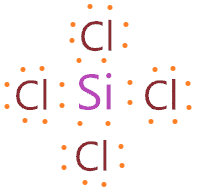2. Find the least electronegative atom and placed it at center

In the lewis diagram, the least electronegative atoms always take the place of the central position because they are more prone to share more electrons than the high electronegative atom. As the central atom always bonded with surrounding atoms, so, it has to share more electrons.

So, in the case of SiCl4, from silicon and chlorine, silicon(1.8) is less electronegative than chlorine(3.16), as electronegativity increases from left to right across a period in the periodic table.

So, put the silicon in the central position and spread all four atoms of chlorine around it.3. Connect outer atoms to central atom with a single bond

In this step, just connect all outer atoms(chlorine) to the central atom(silicon) with a single bond.Now count the valence electrons we have used for making the above structure. As we see, in the above structure, there are 4 single bonds used to connect all chlorine atoms(outer) to the silicon atom(central).

One single bond contains two valence electrons, hence, we used a total of (4 single bonds × 2) = 8 valence electrons from 32 available valence electrons for drawing the SiCl4 lewis structure.

(32 – 8) = 24 valence electrons

Therefore, we are left with 24 valence electrons more.

4. Place remaining valence electrons starting from outer atom first

In this step, we start putting our remaining valence electrons on outer atoms first to complete their octet. So, here, chlorine is the outer atom and each chlorine needs 8 electrons to complete the octet.

So, just put the remaining valence electron on each chlorine atom till they satisfy their octet.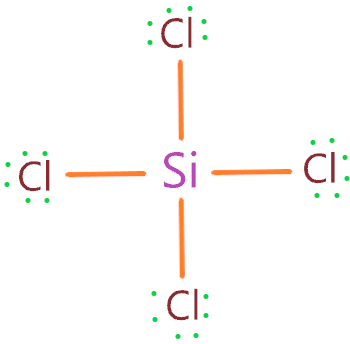### SiCl4 lewis structure

As you see in the above structure, we put the 6 valence electron as dots around each chlorine atom, and these chlorine atoms already sharing the two electrons with the help of a single bond.

Hence, all chlorine atoms completed their octet comfortably as each one has 8 electrons for sharing. Also, our central atom(silicon) also completed its octet as it has 4 single bond connected that contains 8 electrons to share.

We had 24 valence electrons left and in the above structure, we used (6 valence electrons × 4 chlorine atoms) = 24 valence electrons.

∴ (24 – 24) = 0 valence electrons left

So, we have used all valence electrons(32) that are available for drawing the lewis structure of Silicon tetrachloride.

Now just we need to calculate the formal charge of the SiCl4 molecule to verify the stability of the above structure.

5. Check the stability with the help of a formal charge concept

The structure with the formal charge close to zero or zero is the best and stable lewis structure.

To calculate the formal charge on an atom. Use the formula given below-

⇒ Formal charge = (valence electrons – lone pair electrons –  1/2shared pair electrons)

We will calculate the formal charge on the 4th step structure to verify its stability.

For Silicon atom –

⇒ Valence electron of silicon = 4

⇒ Lone pair electrons on silicon = 0

⇒ Shared pair electrons around silicon = 8

F.C. on silicon atom = (4 – 0 – 8/2) = 0

For chlorine atom –

⇒ Valence electron of chlorine = 7

⇒ Lone pair electrons on chlorine = 6

⇒ Shared pair electrons around chlorine = 2

F.C. on chlorine atom = (7 – 6 – 2/2) = 0

∴ Each atom (chlorine and silicon) in the SiCl4 lewis structure gets the formal charge zero.Therefore, the above lewis dot structure of SiCl4 is the best and most stable due to having a formal charge on each atom zero.

## What is the molecular geometry of SiCl4?

The molecular geometry of SiCl4 is tetrahedral as all four outer atoms(chlorine) are pushed away in all directions from the central atoms(silicon) because of repelling force occurs in electron pairs around the central atom.

“Tetrahedral means having four faces”.

The four Si-Cl bonds in SiCl4 geometry take the place of the regular tetrahedron corner, hence, its shape really looks like tetrahedral.

According to the VSEPR theory, when a central atom(silicon) is attached to four bonded atoms(chlorine) then an electron pair around the central atom repel each other as a result all corners atoms(chlorines) spread out as much as they can and takes the place where the repulsion is minimum and stability is much better.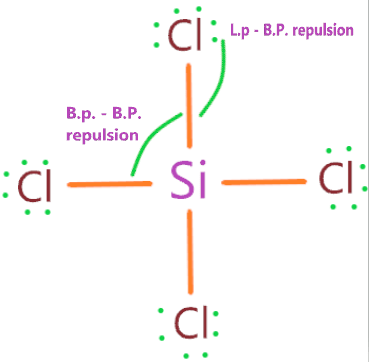As you see in the above structure, the electron pairs(B.P. + B.P. and L.P. + B.P.) repulsion occur, and the surrounding atoms push (adjacent and opposite) atom as much as they can to maximize distance and take the place where repulsion force between these are minimum.

So, all these spreaded atoms hold the place of regular tetrahedron corner, hence we can say, the molecular geometry of SiCl4 is tetrahedral.

If you can’t visualize the molecular geometry of SiCl4, then theoretically we can use an AXN method and VSEPR chart to determines its shape.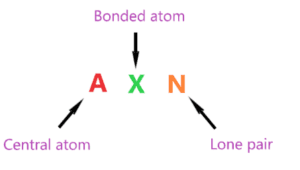That’s how the AXN notation follows as shown in the above picture.

Now we have to find the molecular geometry of SiCl4 by using this method.

AXN notation for SiCl4 molecule:

• A represents the central atom, so as per the SiCl4 lewis structure, silicon is the central atom. A = Silicon
• X represents the bonded atoms, as we know, silicon is making four bonds with chlorine atoms. Therefore, X = 4
• N represents the lone pair on the central atom, silicon atom has zero lone pair on it. Hence, N = 0

So, the AXN notation for the SiCl4 molecule becomes AX4N0 or AX4.

So, as per the VSEPR chart, if the central atom of a molecule contains 0 lone pairs and is cornered by four surrounding atoms, then the molecular shape of that molecule is tetrahedral in nature.

Hence, the molecular shape or geometry for SiCl4 is tetrahedral.### SiCl4 molecular geometry

The electron geometry for SiCl4 is also tetrahedral.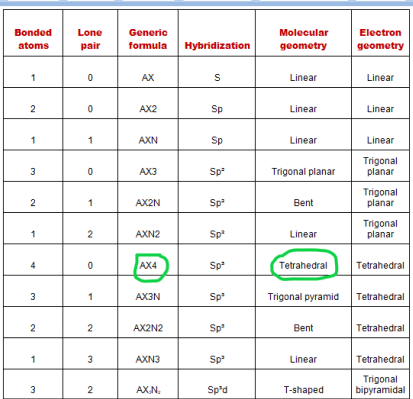## Hybridization of SiCl4

According to hybridization, “two or more orbitals overlap each other and form two or more hybrid orbitals which have same energy and shape”.

To determine the hybridization of any molecule, we have to first determine the hybridization number or the steric number of the central atom.

Note: Steric number and hybridization number is the same.

Steric number is the number of atoms bonded to a central atom of a molecule plus the number of lone pairs attached to the central atom”

∴ Steric number of SiCl4 = (Number of bonded atoms attached to silicon + Lone pair on silicon)

As per the lewis structure of SiCl4, the silicon central atom bonded to 4 chlorine atoms and have a zero lone pair on it.

∴ Steric number of SiCl4 = (4 + 0) = 4

 Steric number Hybridization 1 S 2 Sp 3 Sp² 4 Sp³ 5 Sp³d 6 Sp³d²

So, for a steric number of four, we get the Sp3 hybridization on the SiCl4 molecule.

## The bond angle of SiCl4

“A bond angle is the angle between two atoms in a molecule”.

The bond angle of SiCl4 is 109.5° as the shape of its tetrahedral in nature and as per the VSEPR theory, a regular tetrahedral molecule holds that bond angle of 109.5º.

So, the bond angle of Cl – Si – Cl = 109.5°.## Silicon tetrachloride polarity: is SiCl4 polar or nonpolar?

A polar molecule is asymmetrical contains lone pair and has some dipole moment whereas non-polar molecules are highly symmetrical contain no unshared electrons and have net dipole moment zero.

So, Is SiCl4 polar or nonpolar? SiCl4 is a nonpolar molecule in nature as its shape is highly symmetrical, also its central atom doesn’t contain any lone pair, hence distortion of shape doesn’t happen.

If you see the molecular geometry of SiCl4, all four chlorine atoms are equally spaced around the silicon atom in a tetrahedron corner. Hence, the dipole moment generated along the bond can be easily canceled out, leaving this molecule nonpolar in nature with net dipole moment zero.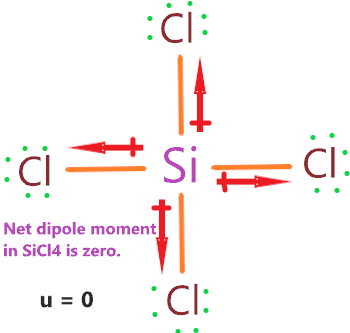Dipole moment generated along with the bond(Si-Cl) due to the separation of charge induced on atoms, this charge is induced because the electronegativity of chlorine is 3.16 and for silicon, it is 1.90. The difference of the electronegativity between these atoms high, this makes a Si-Cl polar covalent bond in nature.

Although the bonds within a molecule(SiCl4) are polar in nature but the structure of SiCl4 is highly symmetrical, this causes a uniform charge distribution in the whole molecule.

Hence, canceling dipole in SiCl4 becomes a lot easy leaving this molecule nonpolar in nature.

## FAQ

### Why is the molecular geometry of SiCl4 is same as its electron geometry?

The molecular geometry of SiCl4 is tetrahedral and its electron geometry is also tetrahedral because as per VSEPR theory, molecular shape considers only bond pairs or atoms while electron geometry considers bonded atoms as well as lone pairs present on the central atom.

According to the lewis structure of SiCl4, the central atom(silicon) doesn’t contain any lone pair on it.

Hence, only bonded atoms are used to determine the geometry of SiCl4.

Therefore, Molecular geometry of SiCl4 = Electron geometry of SiCl4     [∴ no lone pair on central atom of SiCl4]

### How many lone pair and bonded pair electrons a lewis structure of SiCl4 contains?

The central atom(silicon) is attached to the chlorine atoms with four single bonds(bonded pair).

One bonded pair contains two electrons, hence, (4 × 2) = 8 bonded pair electrons present in the lewis structure of Silicon tetrachloride.

Also, lone pair electrons are also called unshared electrons, silicon atoms have no lone pair while each chlorine atom contains 3 lone pairs on it.

Hence, (4 chlorine atoms × 3 lone pairs on each) = 12 lone pairs.

(12 × 2) = 24 lone pair electrons are present in the lewis structure of SiCl4.

## Summary

• The total valence electron is available for the Silicon tetrachloride (SiCl4) lewis structure is 32.
• The hybridization of the SiCl4 molecule is Sp3.
• The bond angle of SiCl4 is 109.5º.
• SiCl4 is nonpolar in nature, although, its bonds are polar.
• The overall formal charge in Silicon tetrachloride is zero.
• Total 24 lone pairs electrons and 8 bonded pairs electrons present in SiCl4 lewis dot structure.
• The molecular geometry of SiCl4 is tetrahedral and electron geometry is also tetrahedral.
##### Subscribe to Blog via Email

Join 2 other subscribers

Share it...